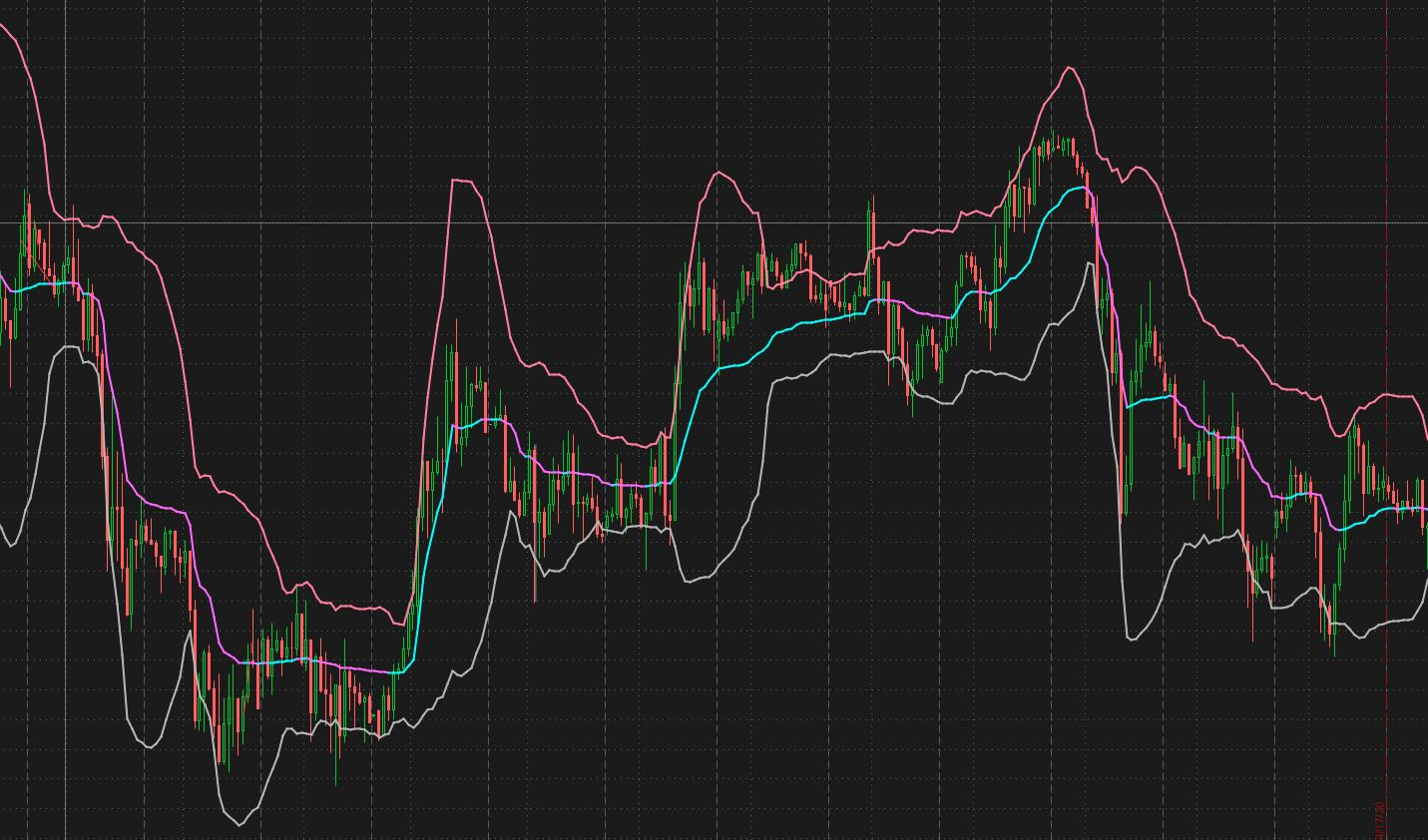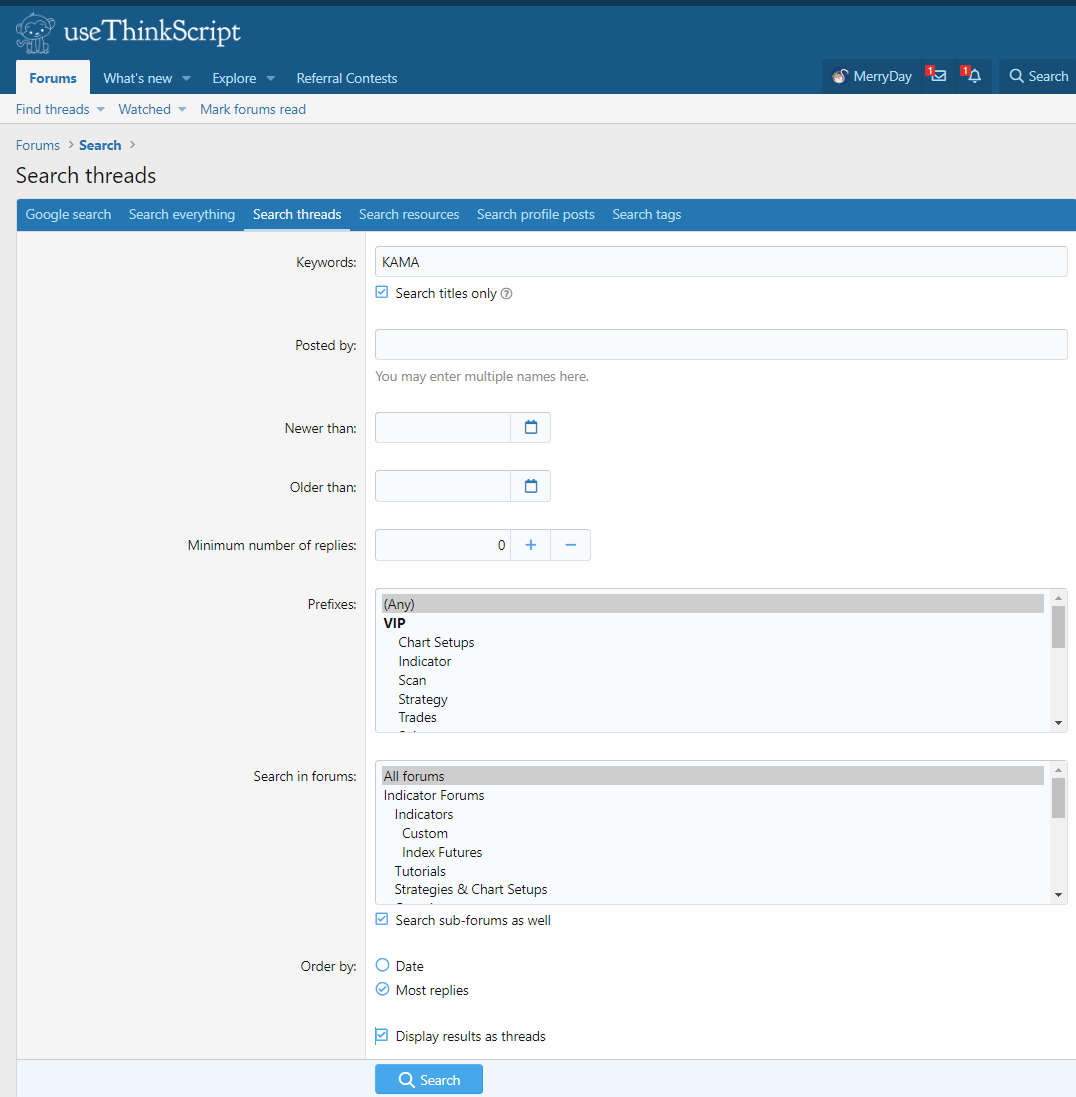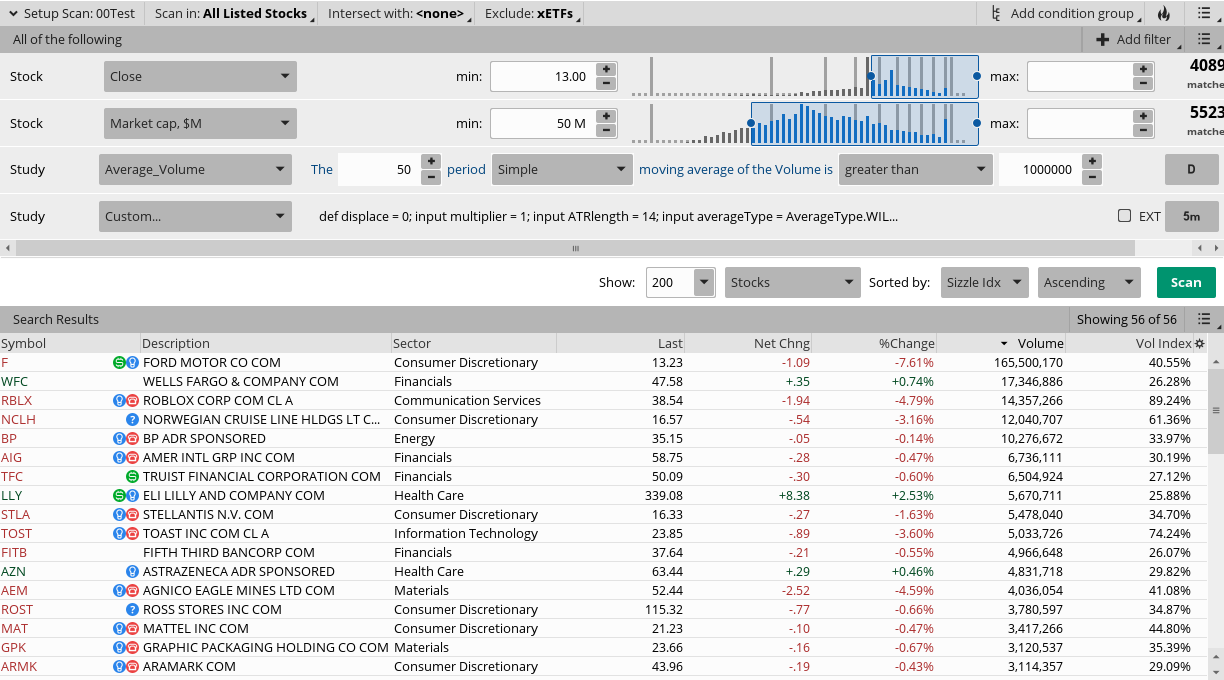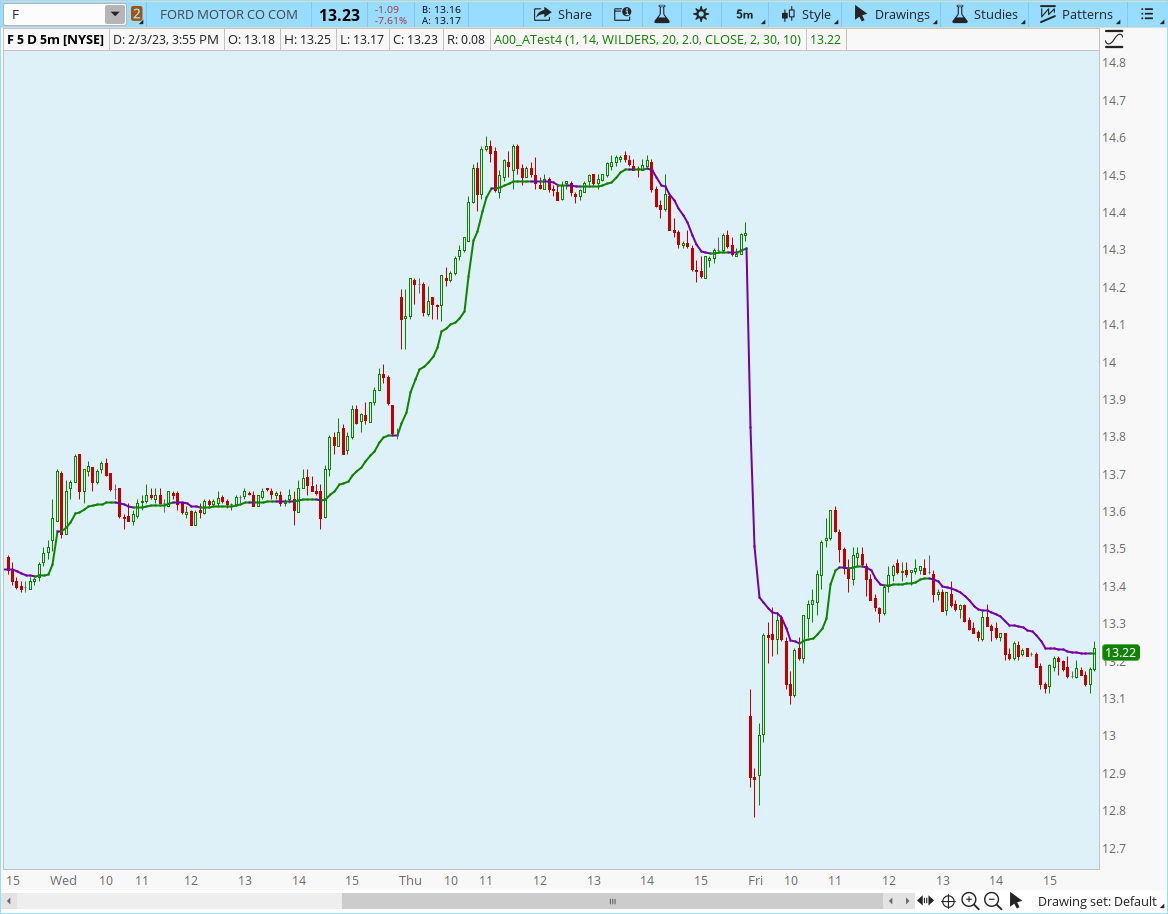# Kaufman's Adaptive Moving Average (KAMA) For ThinkOrSwim

#### BillMurray

##### Member
A buddy of mine wrote this KAMA indicator for ThinkorSwim and a few of us in a circle of friends use it. Austin (who write it) has been trying to massage the code so that it will throw alerts when the trend changes, to be able to scan for trend changes on multiple time frames, and to perhaps put a watchlist column in place to be able to easily pick off tickers changing polarity.

Code:
``````declare lower;
## KAMA (Kaufman Adaptive Moving Average) Settings
input price = close;
input KAMAfastLength = 2;
input KAMAslowLength = 30;
input effRatioLength = 10;
input mode = {default KAMA, AMA};

Assert(KAMAfastLength > 0, "'fast length' must be positive: " + KAMAfastLength);
Assert(KAMAslowLength > 0, "'slow length' must be positive: " + KAMAslowLength);

def direction;
def volatility;
def ER;

switch (mode) {
case KAMA:
direction = AbsValue(price - price[effRatioLength]);
volatility = Sum(AbsValue(price - price), effRatioLength);
ER = if volatility != 0 then direction / volatility else 0;
case AMA:
direction = Double.NaN;
volatility = Double.NaN;
ER = AbsValue((price - Lowest(low, effRatioLength)) -
(Highest(high, effRatioLength) - price)) / (Highest(high,
effRatioLength) - Lowest(low, effRatioLength));
}

def FastSF = 2 / (KAMAfastLength + 1);
def SlowSF = 2 / (KAMAslowLength + 1);
def ScaledSF = ER * (FastSF - SlowSF) + SlowSF;
def AMA = CompoundValue(1, AMA + Sqr(ScaledSF) * (price - AMA),
price);

## EMA Settings
input length = 34;
input displace = 0;

def AvgExp = ExpAverage(price[-displace], length);

##MACD

input fastLength = 12;
input slowLength = 26;
input macdLength = 9;
input averageType = AverageType.EXPONENTIAL;

def diff = reference MACD(fastLength, slowLength, macdLength, averageType).Diff;

## Volume
def Vol = volume(period = AggregationPeriod.DAY);

def avg30dayVol = Average(volume, 30);

def today = volume;
def avg30periodVol = Average(volume, 30);

def BuyVolumeSignal = (today / avg30periodVol) * 100;
def SellVolumeSignal = (today / avg30periodVol) * 100;

def condition1 = price > MovAvgAdaptive and price > AvgExp and BuyVolumeSignal > 15 is true;

def condition2 = price < MovAvgAdaptive and price < AvgExp and BuyVolumeSignal > 15;
;

plot trend = if condition1 is true then 1 else if condition2 >0 then -1 else 0;
trend.SetPaintingStrategy(PaintingStrategy.HISTOGRAM);
trend.DefineColor("Up", Color.UPTICK);
trend.DefineColor("Down", Color.DOWNTICK);
trend.AssignValueColor(if trend > 0 then trend.Color("Up") else if trend < 0 then trend.Color("Down") else GetColor(1));
#Zero.SetDefaultColor(GetColor(5));

Any suggestions would be greatly appreciated, and of course you get to use this. We use it to trade /ES and /NQ futures quite successfully.

Here is a KAMA study which you may enjoy.

Code:
``````input price = close;

#AMA 2,30,10
input fastLength = 2;
input slowLength = 30;
input effRatioLength = 10;
input mode = {default AMA, KAMA};

Assert(fastLength > 0, "'fast length' must be positive: " + fastLength);
Assert(slowLength > 0, "'slow length' must be positive: " + slowLength);

def direction;
def volatility;
def ER;

switch (mode) {
case KAMA:
direction = AbsValue(price - price[effRatioLength]);
volatility = Sum(AbsValue(price - price), effRatioLength);
ER = if volatility != 0 then direction / volatility else 0;
case AMA:
direction = Double.NaN;
volatility = Double.NaN;
ER = AbsValue((price - Lowest(low, effRatioLength)) -
(Highest(high, effRatioLength) - price)) / (Highest(high,
effRatioLength) - Lowest(low, effRatioLength));
}

def FastSF = 2 / (fastLength + 1);
def SlowSF = 2 / (slowLength + 1);
def ScaledSF = ER * (FastSF - SlowSF) + SlowSF;
def AMA = CompoundValue(1, AMA + Sqr(ScaledSF) * (price - AMA),
price);

#KAMA 2,30,10
input fastLength2 = 2;
input slowLength2 = 30;
input effRatioLength2 = 10;
input mode2 = {default KAMA, AMA};

Assert(fastLength2 > 0, "'fast length2' must be positive: " + fastLength2);
Assert(slowLength2 > 0, "'slow length2' must be positive: " + slowLength2);

def direction2;
def volatility2;
def ER2;

switch (mode2) {
case KAMA:
direction2 = AbsValue(price - price[effRatioLength2]);
volatility2 = Sum(AbsValue(price - price), effRatioLength2);
ER2 = if volatility2 != 0 then direction2 / volatility2 else 0;
case AMA:
direction2 = Double.NaN;
volatility2 = Double.NaN;
ER2 = AbsValue((price - Lowest(low, effRatioLength2)) -
(Highest(high, effRatioLength2) - price)) / (Highest(high,
effRatioLength2) - Lowest(low, effRatioLength2));
}

def FastSF2 = 2 / (fastLength2 + 1);
def SlowSF2 = 2 / (slowLength2 + 1);
def ScaledSF2 = ER2 * (FastSF2 - SlowSF2) + SlowSF2;
def AMA2 = CompoundValue(1, AMA2 + Sqr(ScaledSF2) * (price - AMA2),
price);

#KAMA 5,30,10
input fastLength3 = 5;
input slowLength3 = 30;
input effRatioLength3 = 10;
input mode3 = {default KAMA, AMA};

Assert(fastLength3 > 0, "'fast length3' must be positive: " + fastLength3);
Assert(slowLength3 > 0, "'slow length3' must be positive: " + slowLength3);

def direction3;
def volatility3;
def ER3;

switch (mode3) {
case KAMA:
direction3 = AbsValue(price - price[effRatioLength3]);
volatility3 = Sum(AbsValue(price - price), effRatioLength3);
ER3 = if volatility3 != 0 then direction3 / volatility3 else 0;
case AMA:
direction3 = Double.NaN;
volatility3 = Double.NaN;
ER3 = AbsValue((price - Lowest(low, effRatioLength3)) -
(Highest(high, effRatioLength3) - price)) / (Highest(high,
effRatioLength3) - Lowest(low, effRatioLength3));
}

def FastSF3 = 2 / (fastLength3 + 1);
def SlowSF3 = 2 / (slowLength3 + 1);
def ScaledSF3 = ER2 * (FastSF3 - SlowSF3) + SlowSF3;
def AMA3 = CompoundValue(1, AMA3 + Sqr(ScaledSF3) * (price - AMA3),
price);

#Chart Bubbles
def Upsignal = close crosses above MovAvgAdaptive;
def Downsignal = close crosses below MovAvgAdaptive;

input crossingType = {default above, below};

#plot signal = crosses(close, MovAvgAdaptive, crossingType == CrossingType.above);

#signal.DefineColor("Above", GetColor(6));
#signal.DefineColor("Below", GetColor(5));
#signal.AssignValueColor(if crossingType == CrossingType.above then signal.color("Above") else signal.color("Below"));

#signal.SetPaintingStrategy(if crossingType == CrossingType.above
# then PaintingStrategy.BOOLEAN_ARROW_UP
#else PaintingStrategy.BOOLEAN_ARROW_DOWN);

#Vertical Lines

input alerttext = "!!!!! CLOSE crosses AMA  !!!!!";

input UseAlerts = {false, default true};

input AlertType = {default "BAR", "ONCE", "TICK"};

input AlertSound = {"Chimes", "Ring", default "Bell", "NoSound", "Ding"};

def D2 = close > MovAvgAdaptive;
def D1 = close < MovAvgAdaptive;
def OB = D1;
def OS = D2;
def TLB = !D1 and !D2;
AddLabel(TLB, "      (Close <> AMA)      ", Color.YELLOW);
AddLabel(OS, "      (Close > AMA)      ", Color.UPTICK);
AddLabel(OB, "      (Close < AMA)      ", Color.DOWNTICK);``````

Last edited by a moderator:
•Seb_Astian and SpockLogic
Since the KAMA does such a good job at identifying flat markets perfect for mean reversion strategies, would anyone consider using it as the mid point on a channel?
I'm not sure whether a std. deviation or ATR channel would be more optimal. Thoughts?

I don't have KAMA, but here is one with I had made with HULL using ATR, you or someone else can splice KAMA into this code and add more ATR bands as you wish
Code:
``````input price = close;
input length = 200;
input displace = 0;
input multiplier = 1;
input ATRlength = 14;
input averageType = AverageType.WILDERS;

def ATR = MovingAverage(averageType, TrueRange(high, close, low), length);

def HMALine = MovingAverage(AverageType.HULL, price, length)[-displace];

plot upperband = HMALine + (multiplier * ATR);

plot HMA = HMALine;

plot lowerband = HMALine - (multiplier * ATR);

HMA.DefineColor("Up", GetColor(1));
HMA.DefineColor("Down", GetColor(0));
HMA.AssignValueColor(if HMA > HMA then HMA.color("Up") else HMA.color("Down"));``````

We'll have to see how it performs, but at first glance it looks really nice.I added both Standard Deviation and ATR bands, std dev looks to be the most helpful insofar.
Here's the chart with the indicator,
https://tos.mx/0XzXfah
If you have any ideas on a novel strategy or system with it, please let me know. I think it's going to be most helpful in gauging market state, but I might test classic Bollinger bands strategies.

@tradebyday : Thanks for sharing the code.

Is it possible to add custom alerts when the prices breaks the top/bottom?

I am looking to use it as a mean reversion scalp. Will fade the move only when it breaches the top or bottom line.

Coding is really not my thing which is why I am "retired" by the algos.

•turfnerd6300
@tradebyday : Thanks for sharing the code.

Is it possible to add custom alerts when the prices breaks the top/bottom?

I am looking to use it as a mean reversion scalp. Will fade the move only when it breaches the top or bottom line.

Coding is really not my thing which is why I am "retired" by the algos.

Check some other thinkscripts in the forums, as I know several have alerts in them which you could simply copy pasta that portion of code then simply edit the text to match your required criteria. I don't use alerts for anything so it is not my particular expertise to add

Check some other thinkscripts in the forums, as I know several have alerts in them which you could simply copy pasta that portion of code then simply edit the text to match your required criteria. I don't use alerts for anything so it is not my particular expertise to add

Thanks for the quick response. I will do that. Have a awesome day of tradingHey was looking in the search for a simple KAMA indicator and couldnt seem to find it... anyone have code for it?

Thanks

Hey was looking in the search for a simple KAMA indicator and couldnt seem to find it... anyone have code for it?

ThanksLast edited:
Is there a KAMA scanner to filter those stocks which price crossed the 30 KAMA?

Is there a KAMA scanner to filter those stocks which price crossed the 30 KAMA?
Ruby:
``````def displace = 0;
input multiplier = 1;
input ATRlength = 14;
input averageType = AverageType.WILDERS;
input Dev_length = 20;
input Num_Dev = 2.0;
input price = close;
input fastLength = 2;
input slowLength = 30;
input effRatioLength = 10;

def    direction = AbsValue(price - price[effRatioLength]);
def    volatility = Sum(AbsValue(price - price), effRatioLength);
def    ER = if volatility != 0 then direction / volatility else 0;
def FastSF = 2 / (fastLength + 1);
def SlowSF = 2 / (slowLength + 1);
def ScaledSF = ER * (FastSF - SlowSF) + SlowSF;
def AMA = CompoundValue(1, AMA + Sqr(ScaledSF) * (price - AMA),
price);

plot scan = close crosses above MovAvgAdaptive ;``````A buddy of mine wrote this KAMA indicator for ThinkorSwim and a few of us in a circle of friends use it. Austin (who write it) has been trying to massage the code so that it will throw alerts when the trend changes, to be able to scan for trend changes on multiple time frames, and to perhaps put a watchlist column in place to be able to easily pick off tickers changing polarity.

Code:
``````declare lower;
## KAMA (Kaufman Adaptive Moving Average) Settings
input price = close;
input KAMAfastLength = 2;
input KAMAslowLength = 30;
input effRatioLength = 10;
input mode = {default KAMA, AMA};

Assert(KAMAfastLength > 0, "'fast length' must be positive: " + KAMAfastLength);
Assert(KAMAslowLength > 0, "'slow length' must be positive: " + KAMAslowLength);

def direction;
def volatility;
def ER;

switch (mode) {
case KAMA:
direction = AbsValue(price - price[effRatioLength]);
volatility = Sum(AbsValue(price - price), effRatioLength);
ER = if volatility != 0 then direction / volatility else 0;
case AMA:
direction = Double.NaN;
volatility = Double.NaN;
ER = AbsValue((price - Lowest(low, effRatioLength)) -
(Highest(high, effRatioLength) - price)) / (Highest(high,
effRatioLength) - Lowest(low, effRatioLength));
}

def FastSF = 2 / (KAMAfastLength + 1);
def SlowSF = 2 / (KAMAslowLength + 1);
def ScaledSF = ER * (FastSF - SlowSF) + SlowSF;
def AMA = CompoundValue(1, AMA + Sqr(ScaledSF) * (price - AMA),
price);

## EMA Settings
input length = 34;
input displace = 0;

def AvgExp = ExpAverage(price[-displace], length);

##MACD

input fastLength = 12;
input slowLength = 26;
input macdLength = 9;
input averageType = AverageType.EXPONENTIAL;

def diff = reference MACD(fastLength, slowLength, macdLength, averageType).Diff;

## Volume
def Vol = volume(period = AggregationPeriod.DAY);

def avg30dayVol = Average(volume, 30);

def today = volume;
def avg30periodVol = Average(volume, 30);

def BuyVolumeSignal = (today / avg30periodVol) * 100;
def SellVolumeSignal = (today / avg30periodVol) * 100;

def condition1 = price > MovAvgAdaptive and price > AvgExp and BuyVolumeSignal > 15 is true;

def condition2 = price < MovAvgAdaptive and price < AvgExp and BuyVolumeSignal > 15;
;

plot trend = if condition1 is true then 1 else if condition2 >0 then -1 else 0;
trend.SetPaintingStrategy(PaintingStrategy.HISTOGRAM);
trend.DefineColor("Up", Color.UPTICK);
trend.DefineColor("Down", Color.DOWNTICK);
trend.AssignValueColor(if trend > 0 then trend.Color("Up") else if trend < 0 then trend.Color("Down") else GetColor(1));
#Zero.SetDefaultColor(GetColor(5));

Any suggestions would be greatly appreciated, and of course you get to use this. We use it to trade /ES and /NQ futures quite successfully.

Can you please explain the logic? as MACD calculated in the code does not appear to be used at all.

Can you please explain the logic? as MACD calculated in the code does not appear to be used at all.
You are correct. The definition of MACD is extraneous. Feel free to remove it.

### Not the exact question you're looking for?

87k+ Posts
248 Online## The Market Trading Game Changer

Join 2,500+ subscribers inside the useThinkScript VIP Membership Club
• Exclusive indicators
• Proven strategies & setups
• Private Discord community
• Exclusive members-only content
• 1 full year of unlimited support

What is useThinkScript?

useThinkScript is the #1 community of stock market investors using indicators and other tools to power their trading strategies. Traders of all skill levels use our forums to learn about scripting and indicators, help each other, and discover new ways to gain an edge in the markets.

How do I get started?

We get it. Our forum can be intimidating, if not overwhelming. With thousands of topics, tens of thousands of posts, our community has created an incredibly deep knowledge base for stock traders. No one can ever exhaust every resource provided on our site.

If you are new, or just looking for guidance, here are some helpful links to get you started.

What are the benefits of VIP Membership?